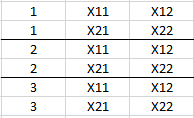# Learning basics of tensors (Chapter 4 of fastbook)

Hi I am on Chapter 4 of the fastbook which discusses the MNIST databases and introduces tensors. Say I have a rank 3 tensor that has a shape of (3,2,2), with the first digit in the shape representing the number of images. So is it accurate to say I have 3 distinct 2x2 matrices?Yes and no.
Yes, you could refer to this as three 2x2 matrices, and it is technically correct.
However, in PyTorch, a Tensor usually has the shape B x C x H x W where B is the batch size, C represents the number of channels/features, and H and W the height and width of the matrix. So if you had three 50x50 px images in greyscale, the Tensor would have a shape of 3 x 1 x 50 x 50. A color image with RGB channels would then be 3 x 3 x 50 x 50.

1 Like

Ok, so since the MNIST images are grey scale and 28x28 pixel images my tensor would be (B x 1 x 28 x 28)? I left B as a variable purposely.

Yes exactly. If you then pass them through the network, the channel/feature dimension will change, representing the different feature maps, generated by the convolutional layers.

1 Like